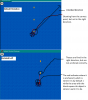# [Solved] How do I Make a projectile fire from a specific point on an object that can rotate?

A

#### AnVee20XX

##### Guest
I lurked a bit on these forums and on the steam forums and have been unable to find a working solution for my case despite may other similar systems.

Put simply, I have an object that the player con rotate. Said object is only supposed to fire projectiles from a specific point. {(18,-8) The main object is 64x64} I can rotate the main object just fine however, I am unable to cause the projectile to fire from anywhere other than where 18,-8 is when the object has not rotated.
How can I make it so that the projectile will always be fired from that area on the sprite as well as make it fire in the direction the sprite is facing?

Attached is a picture of the ship and bullet, including: where the bullet is anchored, how the ship is rotated at the moment, what direction I want the bullet to go.#### flyingsaucerinvasion

##### Member
Hi. If you'd like me to explain how this code works I can, but at the moment I'll just leave it like this:

var _xoffset = 18;
var _yoffset = -8;
var _c = dcos( image_angle );
var _s = dsin( image_angle );
var _barrel_x = x + _c * _xoffset + _s * _yoffset;
var _barrel_y = y + _c * _yoffset - _s * _xoffset;

The present location of the "barrel" of your gun is computed by the above code.
I am assuming here that image_angle is the present rotation of your instance.
you would then create the bullet at (_barrel_x, _barrel_y).

A

#### AnVee20XX

##### Guest
Hi. If you'd like me to explain how this code works I can, but at the moment I'll just leave it like this:

var _xoffset = 18;
var _yoffset = -8;
var _c = dcos( image_angle );
var _s = dsin( image_angle );
var _barrel_x = x + _c * _xoffset + _s * _yoffset;
var _barrel_y = y + _c * _yoffset - _s * _xoffset;

The present location of the "barrel" of your gun is computed by the above code.
I am assuming here that image_angle is the present rotation of your instance.
you would then create the bullet at (_barrel_x, _barrel_y).
Thanks for the help, it anchors like it's supposed to, but I was unable to get it to shoot in the direction the object it was facing. Luckily, due to my failure, I spent some time trying to figure out how to get the bullet to fire in the correct direction. While I was unable to solve this, I did come across an interesting bit of behavior, which I later turned into an enemy object, though its hit detection is not implemented yet.

Here's the mess of code that made this enemy, as well as a video showcasing it and the player character. (To reiterate, I was able to anchor it thanks to you, but am not able to make fire in the direction the character sprite is facing, likely because I'm overlooking something obvious.)

Code:
``with(instance_create(barrel_x-5,barrel_y,obj_EnemyBullet_1)) direction = other.image_angle +15 image_angle = other.image_angle +15;``

P.P.S. I hadn't realized that Trig was in Game Maker, it's kind of neat.

#### NicoFIDI

##### Member
If you are using Code then.
Code:
``````var bullet = instance_create(bullet_x,bullet_y,obj_bullet);
bullet.speed=bullet_speed;
billetes.direction = image_angle;``````

A

#### AnVee20XX

##### Guest
Thanks to both of you, I was able to solve my problem! BTW, I gave you both credit for helping in the description of the video on YouTube.
The final zombie code used in the video can be found just below it.

Code:
``````var _xoffset = 17;
var _yoffset = -9;
var _c = dcos( image_angle );
var _s = dsin( image_angle );

barrel_x = x + _c * _xoffset + _s * _yoffset;
barrel_y = y + _c * _yoffset - _s * _xoffset;

var bullet = instance_create(barrel_x,barrel_y,obj_UserBullet_1);
bullet.direction = image_angle + 45;``````